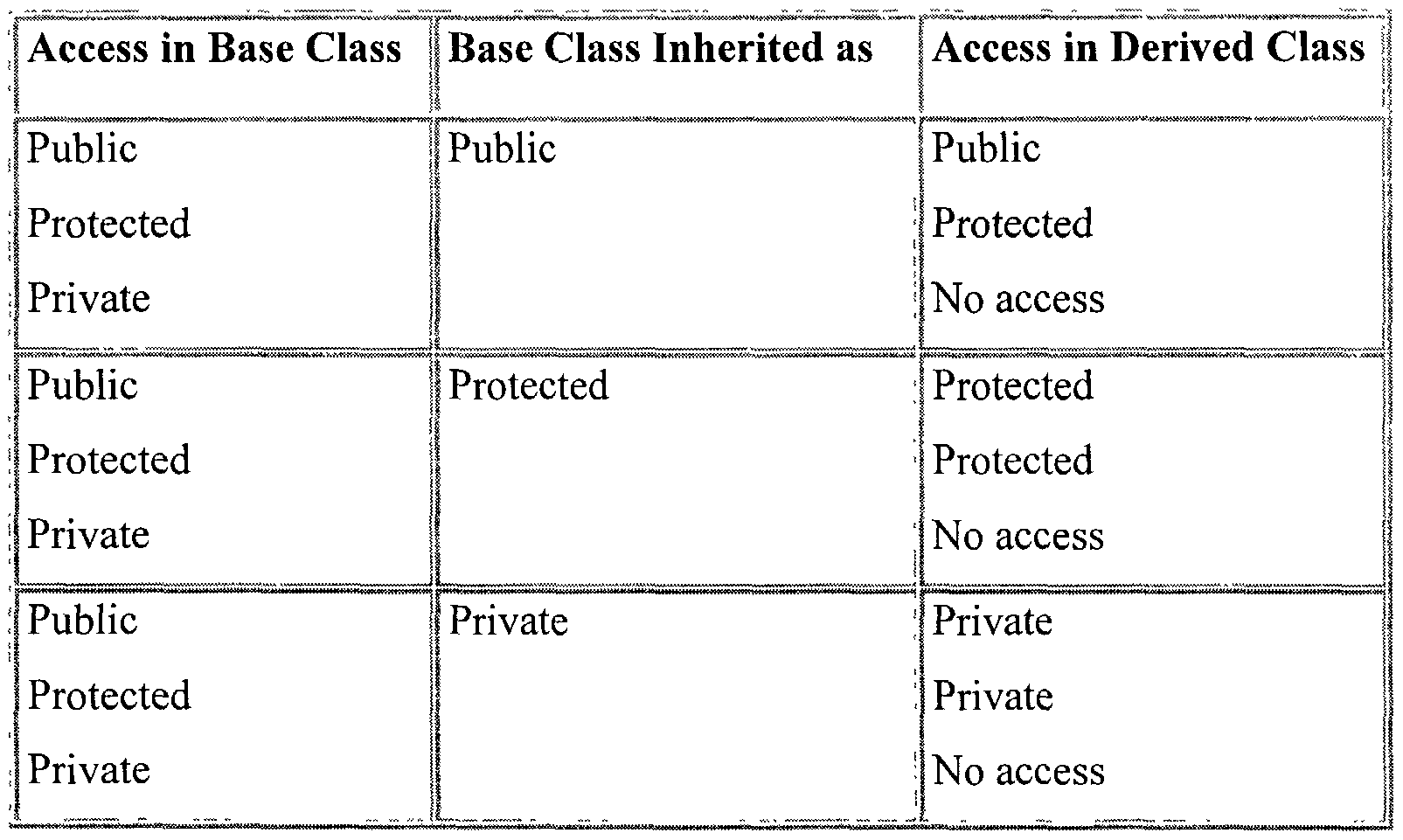# Inheritance in C++

One of the main goals of OOP is the recycling of the classes that you already created. It saves a lot of you time and efforts and makes your code to be more efficient. One of the way to re-use your classes is inheritance. The inheritance is an important concept of OOP. That's why everyone has to understand this concept. Let's start from an example. We have a class man that has that has the following structure
`#include <iostream>using namespace std;class man{public:    man(string _name, int _age, string _sex);    show_man();private:    string name;    int age;    string sex;};`
And you need a class student, that must have the following variables:
`string university; double mark; string profession;`
In this case you have to choose: create a new class student or create a derived class. Use of the inheritance allows you to exclude duplication of code in your program. To declare a derived class you have to use this form of declaration of class: `class derived_class: access_specifier base_class` In our case we will act in the next way:
`class student : public man{public:    student(string _name, int _age, string _sex,string _university,double _mark,string _profession);    show_student();private:    string university;     double mark;     string profession;}`
When you derive a class from base class, private elements of base class are not available for the derived class. You can access them only through interface functions (set and get functions). table of access modes in different types of inheritance:And now we will implement our classes man and student to see how the inheritance can help you to avoid duplication of your code. The man implementation is
`man::man(string _name, int _age, string _sex){    name = _name;    age = _age;    sex = _sex;}int man::show_man(){    cout << "1. Name " << name << endl;    cout << "2. Age " << age << endl;    cout << "3. Sex " << sex << endl;    return 0;}`
it's really simple. The most interesting thing is the implementation of the student class:
`student::student(string _name, int _age, string _sex,string _university,double _mark,string _profession):man(_name, _age,_sex){    university = _university;    mark = _mark;    profession = _profession;}int student::show_student(){    show_man();    cout << "4. From " << university<<endl;    cout << "5. Mark " << mark <<endl;    cout << "6. Profession " << profession << endl;}`
The first line is a good example of the use of inheritance. Here `student::student(string _name, int _age, string _sex,string _university,double _mark,string _profession):man(_name, _age,_sex)` I call the constructor of base class from the constructor of the derived class. In the common view you use the next construction: ` deriverd-class-c-tor(param1,param2,...,paramK,..paramn):base-class-c-tor(param1,param2,...,paramK)` where parameters from #1 to K are common variables of these 2 classes. You can use these classes in your program in this way:
`int main(){    man man1("Peter",27,"M");    cout << "Data of man1" << endl;    man1.show_man();    student student1("John",21,"M","State University of Berlin",8.25,"Engineer");    cout << "Data of student1" << endl;    student1.show_student();    return 0;}`
If you want to use a function from base class in the derived class, you can simply call it by it's name:
`int student::show_student(){    show_man();    //other code}`
Sometimes, the derived and the base class can have the same names of any functions. In this case a conflict between namespaces can appear. For example, We can name the `show_man() ` and ` show_student` with one name `show_info()` In this case we need to use ` :: ` operator. It will look like this:
`int student::show_info(){    man::show_info();    //other code}`
Another important moment is that a class can be derived not only from one base class.If you need to create a class, that contains variables from a number of other classes, you can do it in the following way: `class derived_class: access_specifier1 base_class1,access_specifier2 base_class2,..,access_specifierN base_classN ` For example, you have next classes : wheel, engine, carcass. You need to create class car. You can do it in the following way: ` class car : public wheel,public engine,protected carcass` As a result, the described methods can be used to build a big hierarchy of classes and can be used everywhere.